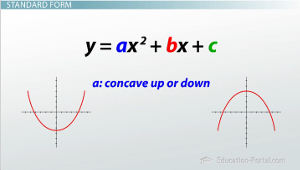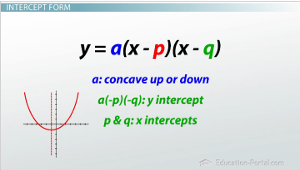# Parabolas in Standard, Intercept, and Vertex Form

An error occurred trying to load this video.

Try refreshing the page, or contact customer support.

Coming up next: Multiplying Binomials Using FOIL and the Area Method

### You're on a roll. Keep up the good work!

Replay
Your next lesson will play in 10 seconds
• 0:06 Kinds of Parabolas
• 1:07 Standard Form
• 2:46 Intercept Form
• 4:26 Vertex Form
• 5:26 Lesson Summary

Want to watch this again later?

Timeline
Autoplay
Autoplay
Speed

#### Recommended Lessons and Courses for You

Lesson Transcript
Instructor: Luke Winspur

Luke has taught high school algebra and geometry, college calculus, and has a master's degree in education.

By rearranging a quadratic equation, you can end up with an infinite number of ways to express the same thing. Learn about the three main forms of a quadratic and the pros and cons of each.

## Kinds of Parabolas

Any time you throw something into the air, it's going to follow a parabolic path. From throwing your wrapper into the trash to throwing a 50-yard touchdown to launching a bird that seems a little bit angry, we see parabolas all over the place. It makes sense then that we want to be able to graph them because the graphs can help us answer questions, like will my bird hit its target?

But as is often the case in math, there is more than one way to go about it. In this case, by simply rearranging the parts of the quadratic equation, we can end up with an infinite number of ways to express the same thing. While most of the ways to write the quadratic equation are redundant and useless, there are three forms that actually have unique uses. These three main forms that we graph parabolas from are called standard form, intercept form and vertex form. Each form will give you slightly different information and have its own unique advantages and disadvantages. In this video, we'll go through both for all the different forms.## Standard Form

Let's begin with standard form, y = ax2 + bx + c. There it is in general form, and here are a few specific examples of what one might look like: y = x2 + x + 1 and y = -4x2 - 5x + 9.

To be completely honest, the main reason this one makes the cut as a useful form is because it's the easiest and most basic to write. While the other forms will require some fancy rearranging with algebra tricks, like factoring or completing the square, most quadratics will be in standard form straight from the beginning. This means that you can dive right into the problem from the get-go, while the other forms will often make you do work before you can even begin. Once we get past that, though, standard form doesn't have too much to offer. Perhaps, its most useful trait is that the a value tells you whether the parabola is concave up (positive a value) or concave down (negative a value), but it turns out that all the forms are going to have this ability.

The second trait of standard form has to do with the y-intercept of the parabola. Since the y-intercept is where x=0, substituting this in shows us that the a and b terms drop out, leaving us with only the c value. Therefore, the c value is always the y-intercept. This is kind of cool, but substituting x=0 into the other forms to find the y-intercept is pretty easy too. The last thing you can do with standard form is calculate the axis of symmetry with the formula x = -b / 2a. Once again, while this is kind of cool, finding the axis of symmetry is possible and actually easier with the other forms.

## Intercept FormThe next form we'll go over is intercept form, y = a(x - p)(x - q). This is the general form, and here are a few specific examples: y = -(x - 1)(x + 5) and y = 3(x + 5)(x + 9).

While it is true that every once in awhile you'll be given a problem that's already in intercept form, it will often be the case that you'll have to first factor the standard-form equation to make it look like intercept form. Although this can sometimes be a headache, there are advantages to doing the work. The a value will, again, tell you whether the parabola is concave up or down, and if you want to find the y-intercept, you can simply substitute in x=0 and quickly evaluate a(-p)(-q).

To unlock this lesson you must be a Study.com Member.

### Register to view this lesson

Are you a student or a teacher?

### Unlock Your Education

#### See for yourself why 30 million people use Study.com

##### Become a Study.com member and start learning now.
Back
What teachers are saying about Study.com

### Earning College Credit

Did you know… We have over 160 college courses that prepare you to earn credit by exam that is accepted by over 1,500 colleges and universities. You can test out of the first two years of college and save thousands off your degree. Anyone can earn credit-by-exam regardless of age or education level.